[BACK]
 Computers, Materials & ContinuaDOI:10.32604/cmc.2021.016097Article

Flower Pollination Heuristics for Parameter Estimation of Electromagnetic Plane Waves

1Department of Electronics, University of Peshawar, Peshawar, 25120, Pakistan
2Future Technology Research Center, National Yunlin University of Science and Technology, Douliou, 64002, Taiwan
3Department of Electrical Engineering, International Islamic University, Islamabad, 44000, Pakistan
5Department of Computer Science and Information, College of Science in Zulfi, Majmaah University, Al-Majmaah, 11952, Saudi Arabia
*Corresponding Author: Muhammad Asif Zahoor Raja. Email: rajamaz@yuntech.edu.tw
Received: 22 December 2020; Accepted: 02 February 2021

Abstract: For the last few decades, the parameter estimation of electromagnetic plane waves i.e., far field sources, impinging on antenna array geometries has attracted a lot of researchers due to their use in radar, sonar and under water acoustic environments. In this work, nature inspired heuristics based on the flower pollination algorithm (FPA) is designed for the estimation problem of amplitude and direction of arrival of far field sources impinging on uniform linear array (ULA). Using the approximation in mean squared error sense, a fitness function of the problem is developed and the strength of the FPA is utilized for optimization of the cost function representing scenarios for various number of sources non-coherent located in the far field. The worth of the proposed FPA based nature inspired computing heuristic is established through assessment studies on fitness, histograms, cumulative distribution function and box plots analysis. The other worthy perks of the proposed scheme include simplicity of concept, ease in the implementation, extendibility and wide range of applicability to solve complex optimization problems. These salient features make the proposed approach as an attractive alternative to be exploited for solving different parameter estimation problems arising in nonlinear systems, power signal modelling, image processing and fault diagnosis.

Keywords: Direction of arrival; flower pollination algorithm; plane waves; parameter estimation

1  Introduction

Parameter estimation specially direction of arrival (DOA) estimation of plane waves plays a vital role in the areas of wireless communication, earthquake, medicine, tracking, navigation, and radio astronomy . In this regard, incorporation of beamforming being adaptive in smart antennas systems gives opportunities to reduce the interferences effects and without using the higher frequency bandwidths, data is transmitted at higher rates. This essential requirement stimulates for the development of algorithms being efficient to estimate DOA. This helps in the determination of complex weights required in beamsteering for preferred direction. Traditional techniques used for estimation of DOA employed the method of periodogram which was based on Fourier transformation. A few of them are conventional beamforming (CBF), Minimum Variance Distortion less Response (MVDR) and dual beamformer. Bartlett, Capon and Lacoss are their developers . The problem with the traditional method was low resolution and further, noise due to Rayleigh limit affected it badly.

To overcome these problems adaptive algorithms were used and methods of maximum likelihood were developed. Stochastic maximum likelihood and deterministic maximum likelihood i.e., SML and DML methods were a few to mention [8,9]. Technique of spatial-temporal processing further improved the accuracy of DML . These methods were having better resolution due to using data model of the received signals completely. Also, these were robust and efficient. But their computational cost is too high due to the multidimensional search and are therefore used occasionally [10,11]. The spectrum-based methods developed in 1980s, were Multiple Signal Classification (MUSIC), Estimation of Signal Parameters via Rotational Invariance Technique (ESPRIT) [12,13]. But the problem with these methods was their computational cost that kept increasing with the increase in the number of array element as they required snapshots at least double in number of the total number of elements in the array. Also, in case of correlated signals, their performance becomes poor. Unitary-ESPRIT method was introduced that was based on unitary transformation and its purpose was to reduce computing cost of ESPRIT method. Conversion of complex covariance matrix into real one reduced its complexity of computation . To rectify the problems in covariance based methods, Direct Data Domain Methods (DDDMs) were developed in mid-nineties. They were based on Matrix Pencil Method (PM) . They were efficient and they required one snapshot in case of DOA to be estimated in real time dynamic conditions.

Techniques, being metaheuristic, have been exploited for the determination of DOA unlike adaptive techniques namely Least Mean Square, MUSIC, ESPRIT and Recursive Least Square etc. due to their effective strength in optimization . To address numerous non-linear problems of constrained optimization in different areas such as optimal energy management, combustion theory fuel ignition model, Magneto-hydrodynamics problems, electromagnetic theory, nano-technology and fractional order systems of non-linear nature , techniques of evolution and swarm intelligence have been applied to them recently.

In this paper, an effective optimization mechanism of flower pollination algorithm (FPA) is employed as a newly introduced algorithm for the parameter estimation of electromagnetic waves of the far field. FPA mimics the process of pollination in flowering plants. FPA is proposed by Yang  and is recently employed in several fields. FPA has impressive nature. Due to this, it has attracted many researchers’ attention in several fields of optimization. Swarm-based optimization technique is used in the FPA with few parameters. The employment of the FPA in various optimization problems has shown a robust performance. Further, FPA being simple optimization method is a flexible, adaptable, and scalable algorithm. FPA gives very beneficial results in solving various optimization problems as compared with other metaheuristic algorithms. These problems are from different areas such as signal and image processing, clustering and classification, electrical systems, wireless networks, computer gaming, travelling salesman problem and others many more . In the present work collective estimation of DOA as well as amplitudes of plane wave (electromagnetic) falling on ULA is considered. To minimize error between desired and actual responses, mean squared error (MSE) is used as a fitness criterion. A single snapshot is required by this fitness function and works well, particularly in the existence of local optima. For substantial statistical analysis of FPA, Monte Carlo simulations (in a large number) are done using MATLAB. For this analysis two, three, and four sources are considered and are investigated for fitness, robustness, MSE, and complexity (computational). Main properties of the proposed mechanism are as follows:

•    Exploitation of pollination based optimization technique FPA for the novel study of DOA estimation

•    Augmented power of FPA is built for the parameter estimation (effectively) of plane waves of sources.

•    The design mechanism is validated for different scenarios of far field sources.

•    The accuracy, robustness, and reliability of the algorithm are proven via results of the statistics in terms of parameters fitness.

•    Ease of implementation, simple in concept, extendibility, handling complex models and wide range of applicability are further advantages of the scheme.

The paper is arranged as follows: In Section 2, plane waves incident on a ULA is given as general data model for parameter estimation, while details about proposed scheme that has foundation on FPA are given in Section 3. Section 4 provides results and discussion on the results. The last section presents the conclusion and future work.

2  General System Model for Parameters Amplitude and DOA Estimation

For model development, consider narrow band sources of EM plane waves P in number. The plane waves are falling on ULA. The ULA has “N” elements. The inter-element spacing is “d” which is uniform between any two consecutive elements. It is portrayed in Fig. 1. For PN, the output of the nth element of ULA is given as:

yn(t)=l=1Pil(t)exp(-j(ξln))+ηn(t).(1)

for n=1,2,,N. For single snapshot, Eq. (1) becomes

yn=l=1Pil exp(-j(ξln))+ηn(2)

In Eq. (2), the value of ξ is kdcosθl which is delay due to propagation between the reference and nth element. Likewise, value of k is 2π/λ and is termed as wave number. Eq. (2) in a vector form is given as,

(y0y1yN-1)=(11e-jkdcosθ1e-jkdcosθpe-jkd(N-1)cosθ1e-jkd(N-1)cosθP)(i1i2iP)+(η0η1ηN-1)(3)

Here θ in above matrix and i in the vector denote angle (elevation) and amplitude of plane waves respectively. The angle “θ” is with respect to broad side. Eq. (3) can be given in compact form as:

y=Si+η(4)

S is the symbol used here for the steering matrix. It has got steering vectors of P sources. AWGN introduced in each antenna element is symbolized here as η. It does not depend upon source waves. The parameters DOA (θ) and amplitudes (i) in Eq. (3) are unknown for the lth source where l ranges from 1 to P.Figure 1: Plane waves falling on ULA antenna

3  Methodology

A new meta heuristic technique called FPA was originally proposed by Yang  in 2012. This algorithm uses the concept of pollination in plants. Pollination is prerequisite of the fertilization in plant species. In this process pollens migrate, meet the pollens of another flower or other plants. The flower may be of the same plant. Likewise, the other plants may be of same species. This results in fruitful fertilization. In biotic pollination, pollinators (insects/birds etc.) are carriers of the pollens from one flower to another. The same are transferred via wind or simple diffusion in abiotic pollination. Most of the part is played by the biotic pollination in nature. Flower constancy for pollination is another responsible factor. In this, pollinators limit themselves with plants of particular type. . The mathematical expression for FPA is written as :

xit+1=xit+L(xit-gbest)(5)

In Eq. (5), L=step size. It is always positive and nonzero and it determines the pollination strength. Step size “L” represents “Levy Flights.” The further necessary details of Eq. (5) can be seen in .

In this work, FPA is developed for parameter estimation of electromagnetic plane waves. The flow chart of FPA is portrayed in Fig. 2. While, the pseudo-code is given as follows: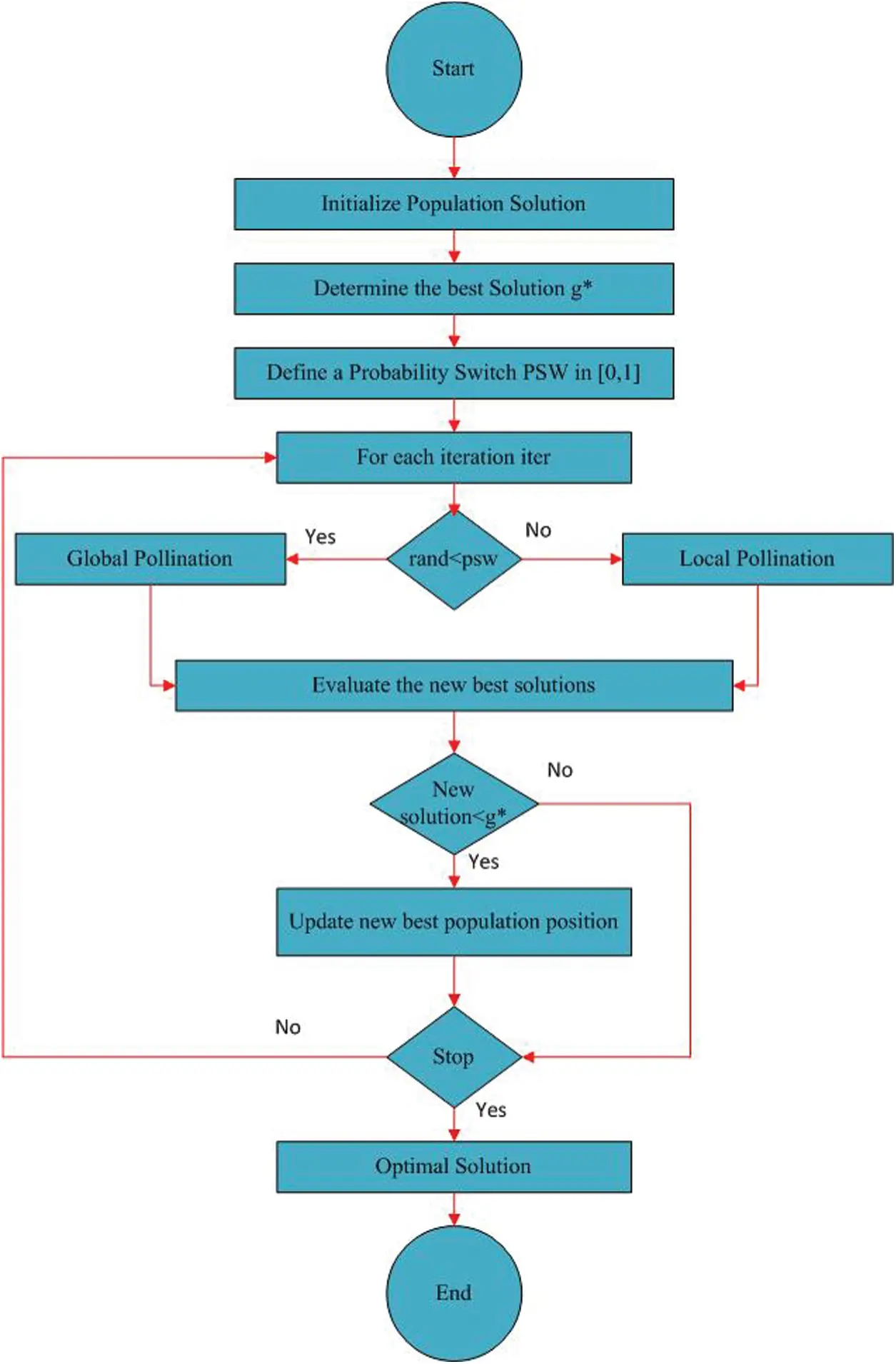Figure 2: Flow chart of FPA

Step 1 Population Initialization

M” individuals are generated randomly with entries equal to decision variable of optimization problem, i.e., DOA estimation of plane waves. The Jth individual representation of FPA is mathematically given as:

Fj=(f1f2fM)=(i^1θ^1i^2θ^2i^Mθ^M)jfor any{i^=(î1,î2,,îP),θ^=(θ^1+P,θ^2+P,,θ^2P).(6)

For the current optimization problem, the constraints associated with are:

{i^R:lbîkubθ^R:0θ^k+Pπ

Amplitude bounds (lower and upper) are symbolized here as lb and ub respectively, and k=1,2,,P. Settings for FPA parameters are done, i.e., number of individuals in population, number of iterations, probability switch, bounds etc.

Step 2 Computation of Fitness

The fitness function is expressed in terms of mean squared error. For noiseless environment it is given as:

ε={1Nn=1N|y(n)-ŷ(n)|2,1Nn=1N|l=1Pil exp(-j(kdncosθP+l))-l=1Pîl exp(-j(kdncosθ^P+l))|2(7)

Fitness is computed for each individual of population F using Eq. (7) and are ranked accordingly.

Step 3 Determine g*, the initial best solution

Step 4 Defines a probability switch psw[0,1].

Step 5 Compute fitness value of all n members/solution/flowers.

Step 6 if rand<psw, then

Step 7 Draw a step vector L (d-dimensional) obeying Levy Distribution

Step 8 Carry out global pollination via xit+1=xit+L(xit-gbest)

Else

Step 9 Draw a uniform distribution ε[0,1]

Step 10 Randomly choose j and k among all solutions

Step 11 Do local pollination via xit+1=xit+ε(xjt-xkt)

Step 12 Evaluate the new best solution

Step 13 If the new solution<g*

Step 14 xt = xt+1

Step 15 Find the current best solution g* among all xit

Step 16 For global best solution, store the parameters, and its fitness for each run.

Step 17 For reliability, Steps 1–16 are repeated for sufficiently huge runs to have a huge set of data.

Step 18 For FPA performance evaluation, the fitness as in Eq. (7) and norm of the absolute error (NAE), also called the Euclidean length, as defined below are used:

v=k=1N|vk|2(8)

where N is index of element in vector v.

4  Results and Discussion

In this section, work is presented in terms of simulations for three scenarios. In each scenario we have two sources model (2SM), three sources model (3SM) and four sources model (4SM). The EM plane wave sources are P where P=2, 3 and 4 respectively. ULA has 4, 6 and 8 elements respectively. The optimization strength of FPA is exploited for parameter estimation of these far field sources. The true 2-D parameters of the sources are written as follows:

S=[i1i2]=[0.5,1]andθ=[θ1θ2]=[0.87266,1.4835],S=[i1i2i3]=[3,1,5]andθ=[θ1θ2θ3]=[1.8326,2.7053,0.87266],S=[i1i2i3i4]=[1,1.5,2,2.5]andθ=[θ1θ2θ3θ4]=[2.618,0.34907,1.0472,1.9199]

The scheme designed (based on FPA) is utilized for parameter estimation. It is employed for both situations (noisy as well as noiseless) as given in the section of methodology. For each scenario, five cases are worked out as: Case 1: 2 SM with no noise and given noise is added for rest of the four cases namely Case 2: 2 SM having 65 dB, Case 3: 2 SM having 55 dB, Case 4: 2 SM having 45dB and Case 5: 2 SM having 35 dB. The results are obtained for 100 independent runs of the FPA. Objective function for any of the scenario is formulated as:

ε=1Nn=1N(|l=1Pil exp-jkd(n-1)cos(θl)+ηn-l=1Pĩl exp-jkd(n-1)cos(θ̃l)|)2(9)

Eq. (9) denotes fitness function. In this ηn denotes noise and its value is 0, 65, 55, 45, and 35dB. The settings of FPA are chosen as: Population size=10, Probability switch=0.8, No. of iterations=10,000, lower bounds of amplitude=0, lower bounds of angle=0, upper bounds of amplitude=10 and upper bounds of angle=pi, dimension size (dim)=2*P.

Table 1: Outcomes of FPA for scenario 1 of two far field sources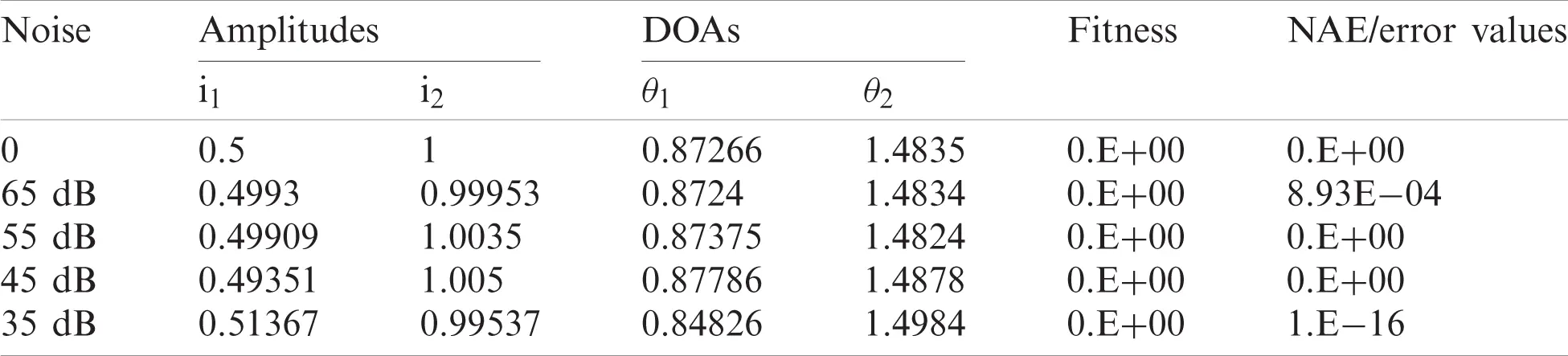Table 2: Outcomes of FPA for scenario 2 of three far field sources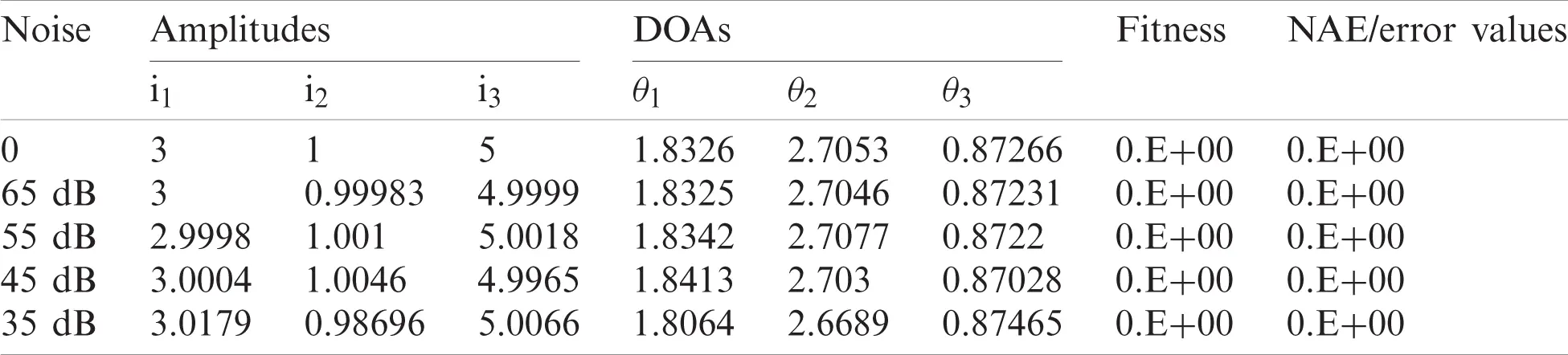Table 3: Outcomes of FPA for scenario 3 of four far field sources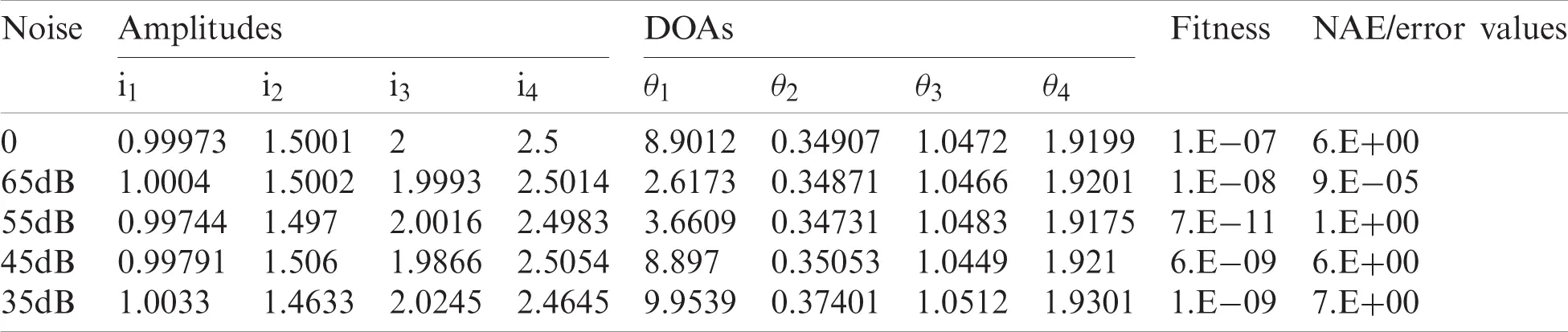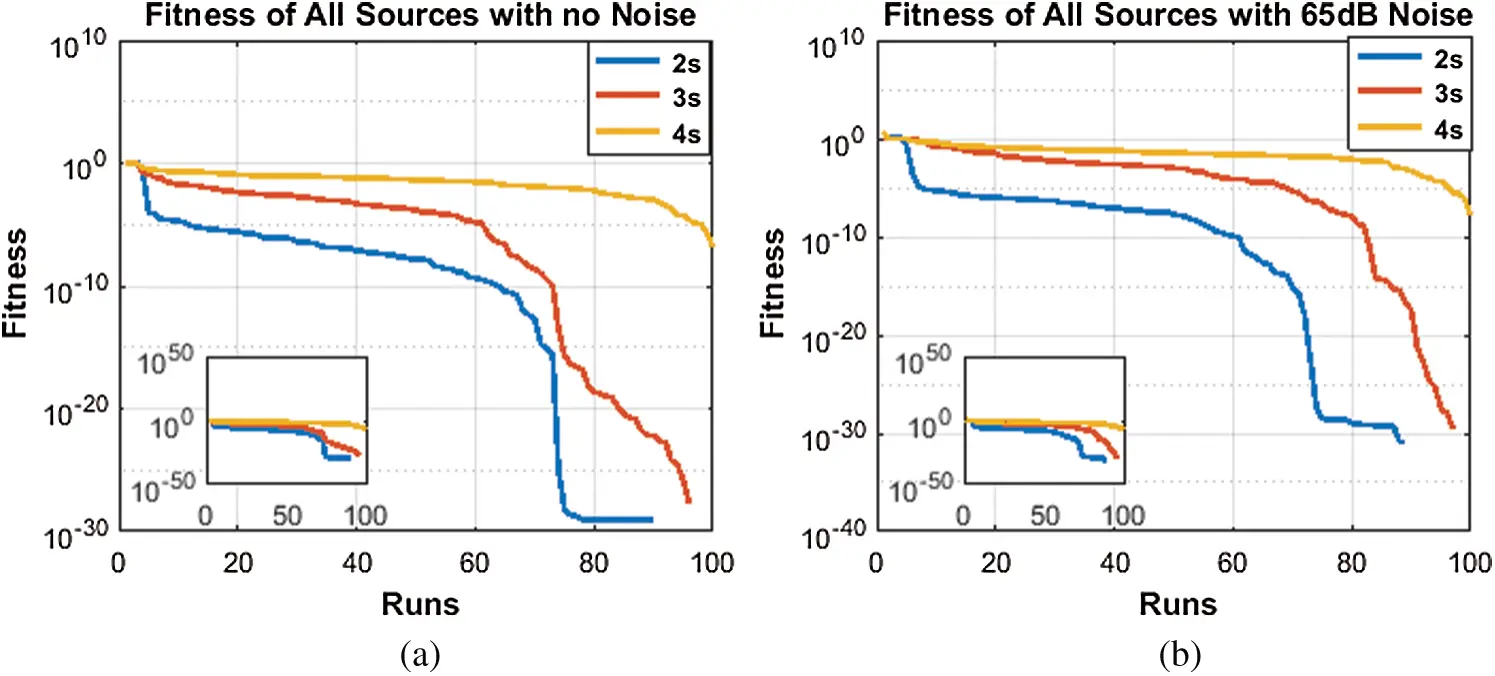Figure 3: Fitness with and without noise. (a) No noise case, (b) 65 dB noise case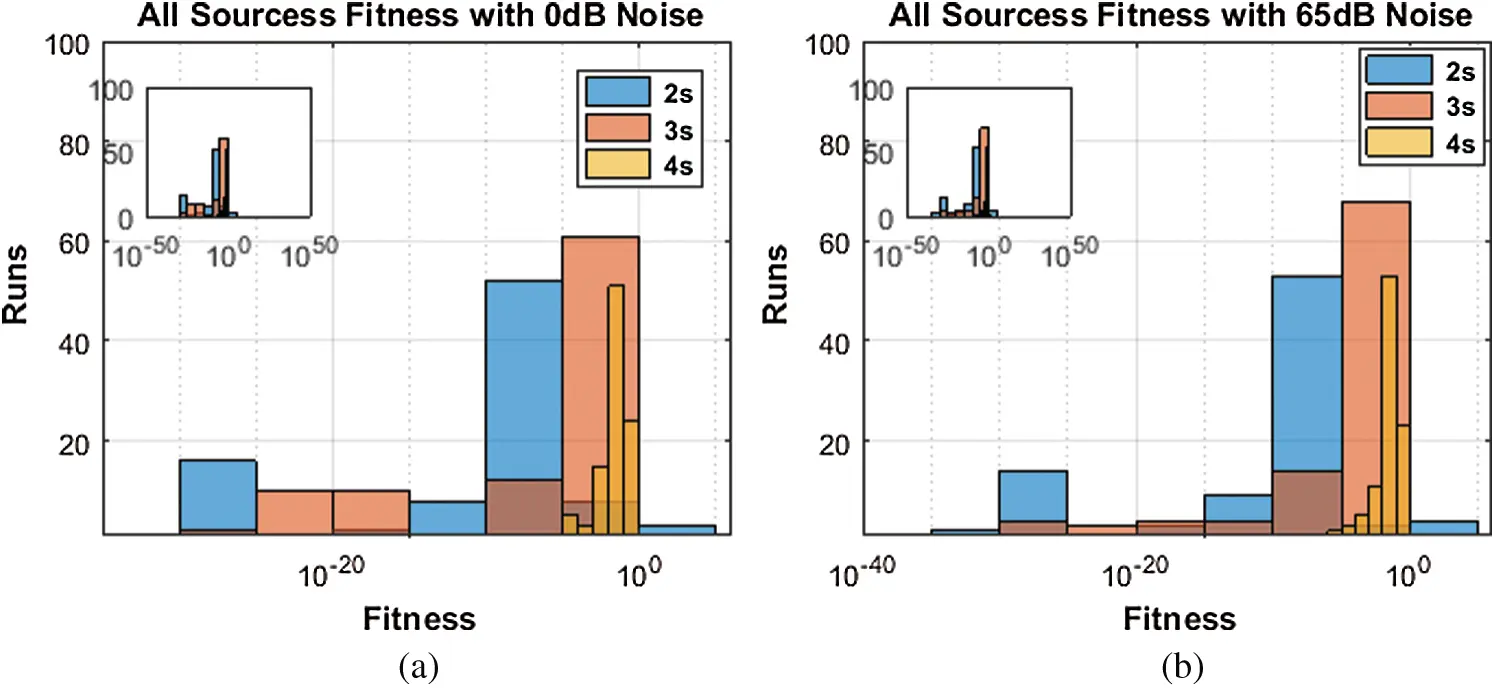Figure 4: Histogram with and without noise. (a)No noise case, (b) 65 dB noise caseFigure 5: CDF with and without noise. (a)No noise case, (b) 65 dB noise case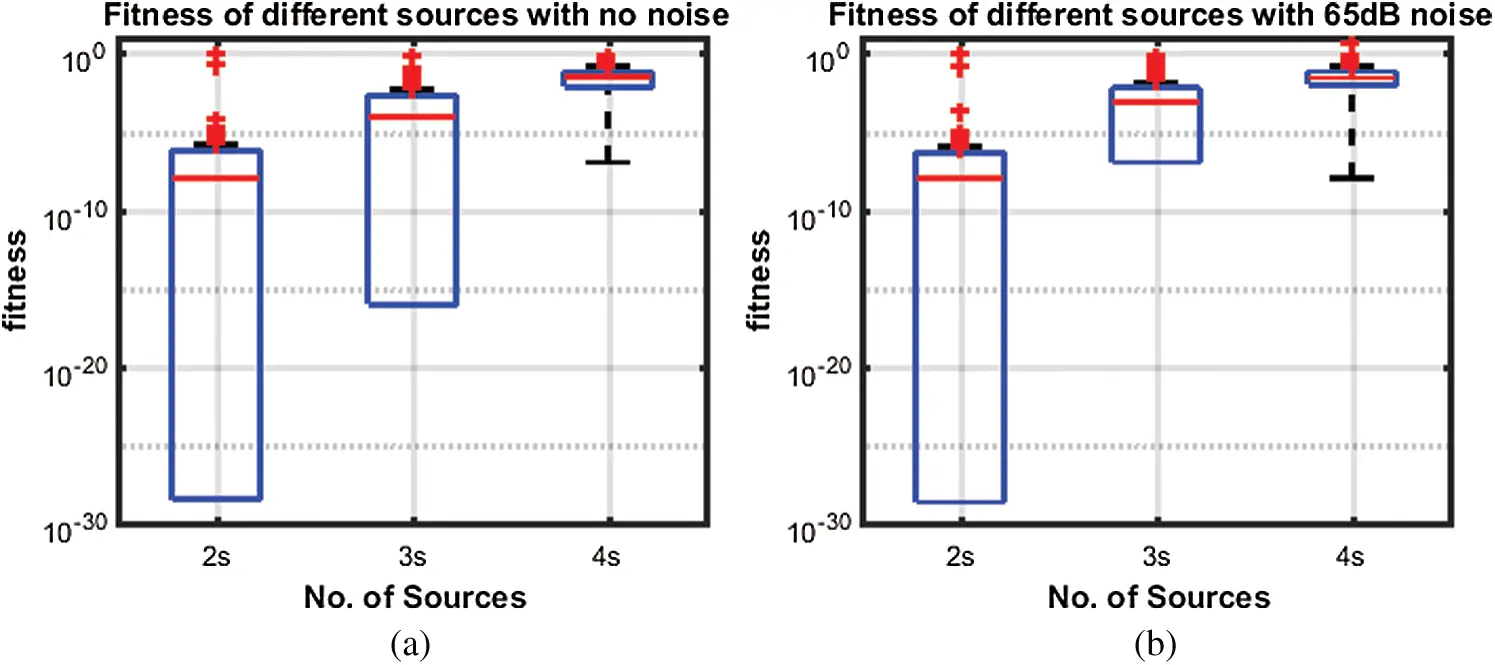Figure 6: Box Plots with and without noise. (a)No noise, case (b)65 dB noise case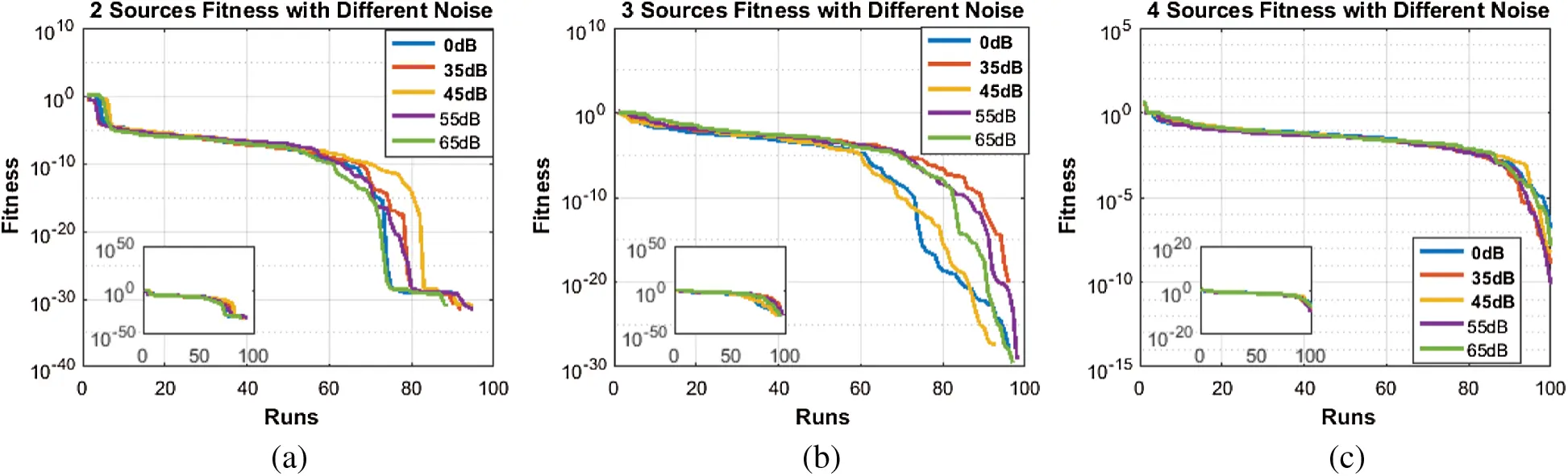Figure 7: Fitness of same source with different noise. (a) 2 sources (b) 3 sources (c) 4 sources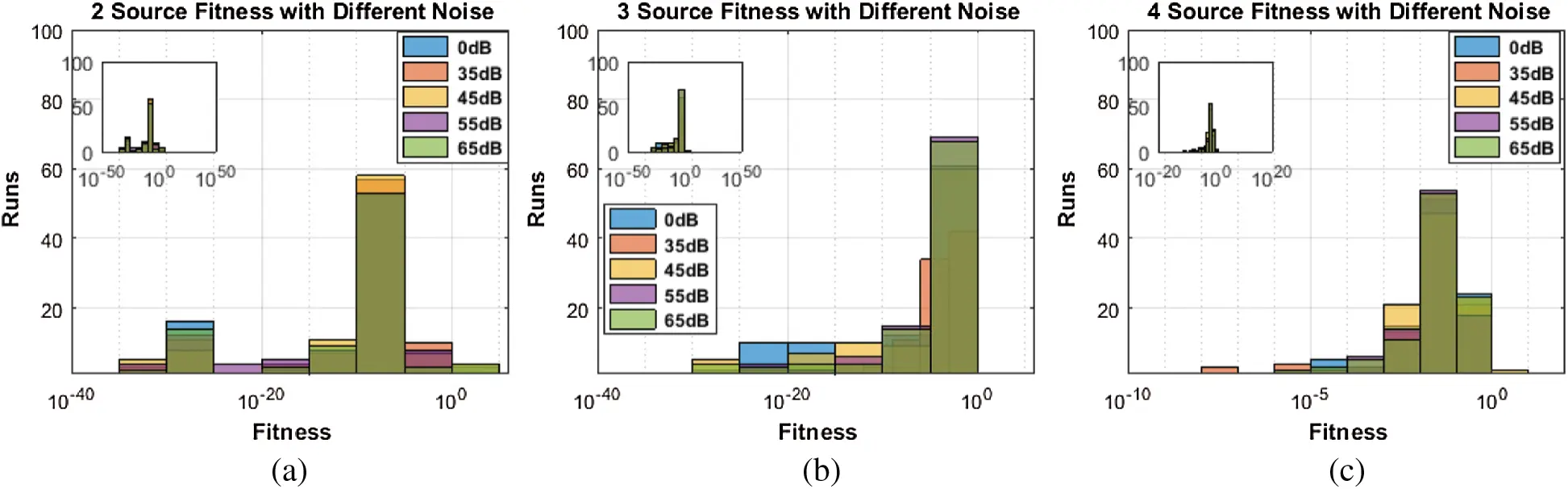Figure 8: Histogram of same source with different noise. (a) 2 sources (b) 3 sources (c) 4 sources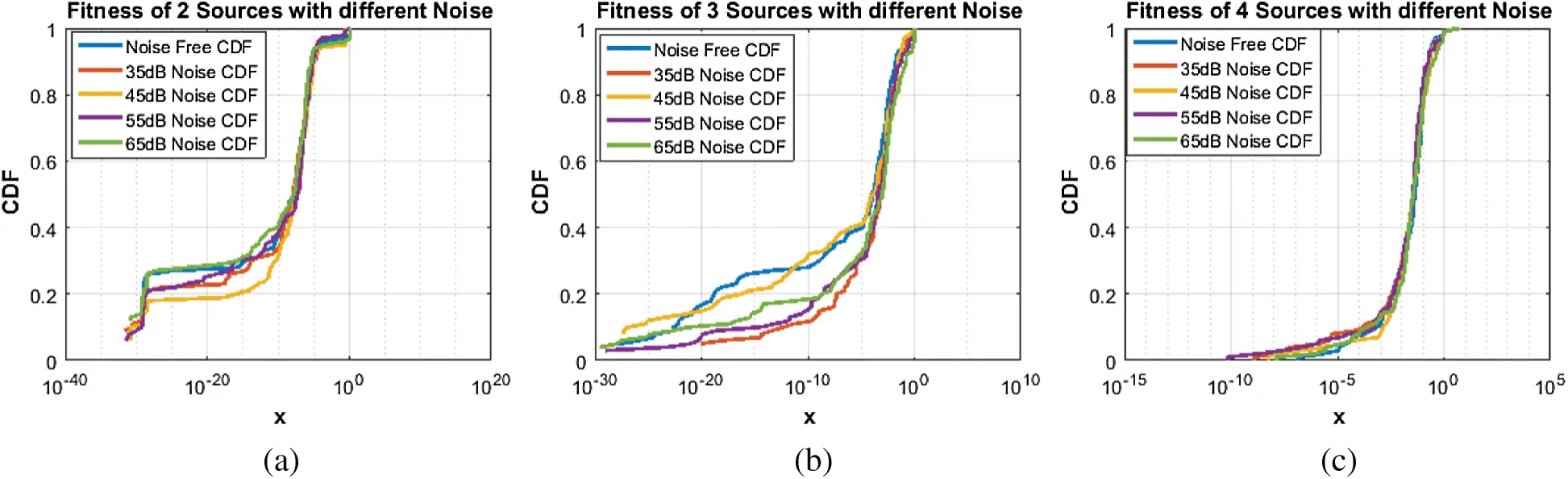Figure 9: CDF of same source with different noise. (a) 2 sources (b) 3 sources (c) 4 sources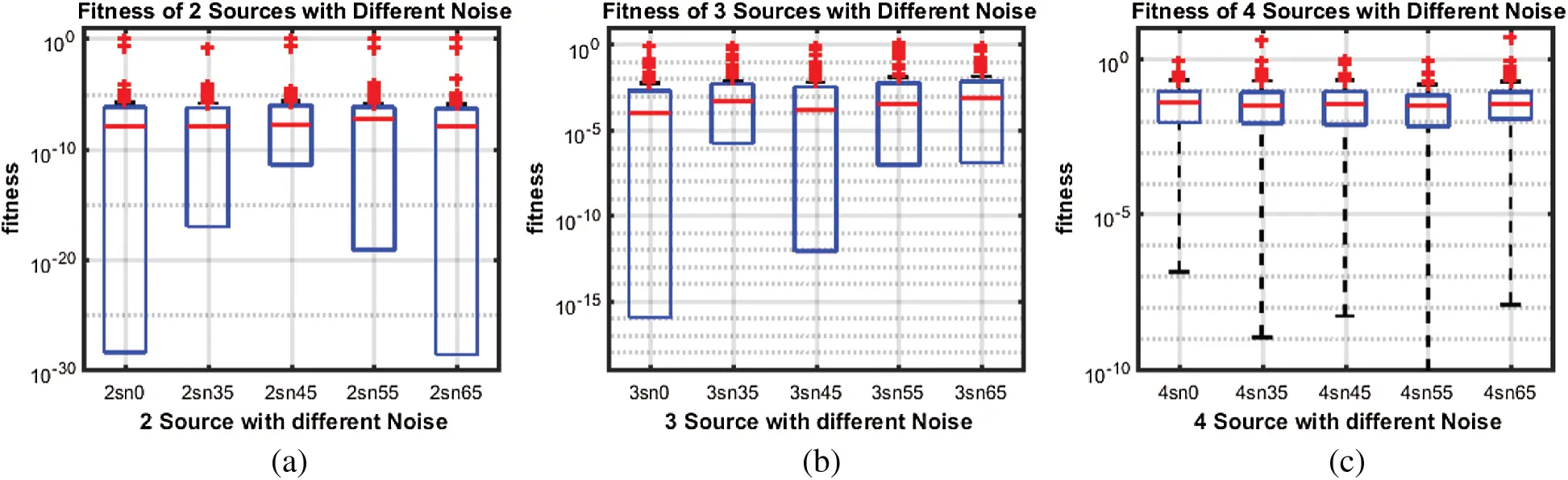Figure 10: Box plot of same source with different noise. (a) 2 sources (b) 3 sources (c) 4 sources

Likewise, all Figs. 710 results show that even in low SNR situation, the proposed algorithm performed well. With low estimation accuracy, particularly in case of two and three sources, it has produced fair enough results. However, its performance is degraded in case of four impinging sources. The reason is clear that as number of sources increases, problem of identification becomes harder.

5  Conclusion

An innovative application of flower pollination heuristic is introduced for reliable parameter estimation of electromagnetic plane waves impinging on antenna array geometries. The accuracy, stability and robustness of the proposed flower pollination heuristic is verified from actual value of system parameter for single and multiple autonomous runs. The worth of the proposed FPA is further established through statistical assessments based on fitness, histograms, cumulative distribution function and box plots analysis for two, three and four source model of DOA parameter estimation in noisy and noiseless environments.

In future, one may exploit the proposed methodology for different optimization problems including power signal estimation , Hammerstein nonlinear system identification , fault diagnosis , travelling salesman problem , second order boundary value problems  and image processing .

Funding Statement: The authors would like to thank the Deanship of Scientific Research at Majmaah University for supporting this work under Project Number No. R-2021-27.

Conflicts of Interest: All the authors of the manuscript declared that there are no potential conflicts of interest.

## References

1. T. E. Tuncer and B. Friedlander, Classical and Modern Direction of Arrival Estimation. Burlington, MA: Elsevier Inc., 2009.
2. C. L. Meng, S. W. Chen and A. C. Chang, “Direction-of-arrival estimation based on particle swarm optimization searching approaches for CDMA signals,” Wireless Personal Communications, vol. 81, no. 1, pp. 343–357, 2015.
3. R. Cao, C. Wang and X. Zhang, “Two-dimensional direction of arrival estimation using generalized ESPRIT algorithm with non-uniform L-shaped array,” Wireless Personal Communications, vol. 84, no. 1, pp. 321–339, 2015.
4. Z. Chen, G. K. Gokeda and Y. Yu, Introduction to Direction-of-Arrival Estimation. Norwood: Artech House, 2010.
5. M. S. Bartlett, “Smoothing periodograms from time series with continuous spectra,” Nature, vol. 161, no. 4096, pp. 686–687, 1948.
6. J. Capon, “High resolution frequency-wavenumber spectrum analysis,” Proceedings of the IEEE, vol. 57, no. 8, pp. 1408–1418, 1969.
7. R. T. Lacoss, “Data adaptive spectral analysis methods,” Geophysics, vol. 36, no. 4, pp. 661–675, 1971.
8. P. Stoica and K. C. Sharman, “Maximum likelihood methods for direction-of-arrival estimation,” IEEE Transactions on Acoustics, Speech, and Signal Processing, vol. 38, no. 7, pp. 1132–1143, 1990.
9. M. I. Miller and D. R. Fuhrmann, “Maximum-likelihood narrow-band direction finding and the EM algorithm,” IEEE Transactions on Acoustics, Speech, and Signal Processing, vol. 38, no. 9, pp. 1560–1577, 1990.
10. L. C. Godara, “Application of antenna arrays to mobile communications. II. Beam-forming and direction-of-arrival considerations,” Proc. of the IEEE, vol. 85, no. 8, pp. 1195–1245, 1997.
11. Z. Ariela and B. Friedlander, “Direction of arrival estimation using parametric signal models,” IEEE Transactions on Signal Processing, vol. 44, no. 2, pp. 339–350, 1996.
12. F. Richard, “Analysis of min-norm and MUSIC with arbitrary array geometry,” IEEE Transactions on Aerospace and Electronic Systems, vol. 26, no. 6, pp. 976–985, 1990.
13. M. Haardt and M. E. Ali-Hackl, “Unitary ESPRIT: How to exploit additional information inherent in the rotational invariance structure,” in ICASSP Proc., Adelaide, Australia, pp. 229–232, 1994.
14. H. Krim and J. G. Proakis, “Smoothed eigenspace-based parameter estimation,” Automatica, vol. 30, no. 1, pp. 27–38, 1994.
15. Y. Hua and T. K. Sarkar, “Matrix pencil method for estimating parameters of exponentially damped-undamped sinusoids in noise,” IEEE Transactions on Acoustics, Speech, and Signal Processing, vol. 38, no. 5, pp. 814–824, 1990.
16. M. Li and Y. Lu, “A refined genetic algorithm for accurate and reliable DOA estimation with a sensor array,” Wireless Personal Communications, vol. 43, no. 2, pp. 533–547, 2007.
17. L. Boccato, R. Krummenauer, R. Attux and A. Lopes, “Application of natural computing algorithms to maximum likelihood estimation of direction of arrival,” Signal Processing, vol. 92, no. 5, pp. 1338–1352, 2012.
18. L. Boccato, R. Krummenauer, R. Attux and A. Lopes, “Improving the efficiency of natural computing algorithms in DOA estimation using a noise filtering approach,” Circuits, Systems, and Signal Processing, vol. 32, no. 4, pp. 1991–2001, 2013.
19. Z. Zhang, J. Lin and Y. Shi, “Application of artificial bee colony algorithm to maximum likelihood DOA estimation,” Journal of Bionic Engineering, vol. 10, no. 1, pp. 100–109, 2013.
20. J. A. Khan, M. A. Z. Raja, M. M. Rashidi, M. I. Syam and A. M. Wazwaz, “Nature-inspired computing approach for solving non-linear singular Emden–Fowler problem arising in electromagnetic theory,” Connection Sciences, vol. 27, no. 4, pp. 377–396, 2015.
21. M. A. Z. Raja, “Solution of the one-dimensional Bratu equation arising in the fuel ignition model using ANN optimised with PSO and SQP,” Connection Science, vol. 26, no. 3, pp. 195–214, 2014.
22. M. A. Z. Raja and R. Samar, “Numerical treatment for nonlinear MHD Jeffery-Hamel problem using neural networks optimized with interior point algorithm,” Neurocomputing, vol. 124, no. 29, pp. 178–193, 2014.
23. H. Xia, H. Chen, Z. Yang, F. Lin and B. Wang, “Optimal energy management, location and size for stationary energy storage system in a metro line based on genetic algorithm,” Energies, vol. 8, no. 10, pp. 11618–11640, 2015.
24. M. A. Z. Raja, U. Farooq, N. I. Chaudhary and A. M. Wazwaz, “Stochastic numerical solver for nanofluidic problems containing multi-walled carbon nanotubes,” Applied Soft Computing, vol. 38, no. 6348, pp. 561–586, 2015.
25. M. A. Z. Raja, M. A. Manzar and R. Samar, “An efficient computational intelligence approach for solving fractional order Riccati equations using ANN and SQP,” Applied Mathematical Modeling, vol. 39, no. 10,11, pp. 3075–3093, 2015.
26. X. S. Yang, “Flower pollination algorithm for global optimization,” in UCNC Lecture Notes in Computer Science, vol. 7445. Berlin, Heidelberg: Springer, pp. 240–249, 2012. https://doi.org/10.1007/978-3-642-32894-7_27.
27. A. Abdelaziz, E. Ali and S. A. Elazim, “Combined economic and emission dispatch solution using flower pollination algorithm,” International Journal of Electrical Power & Energy Systems, vol. 80, no. 4, pp. 264–274, 2016.
28. U. Singh and R. Salgotra, “Synthesis of linear antenna array using flower pollination Algorithm,” Neural Computing and Applications, vol. 29, no. 2, pp. 435–445, 2018.
29. A. Abdelaziz, E. Ali and S. A. Elazim, “Implementation of flower pollination algorithm for solving economic load dispatch and combined economic emission dispatch problems in power systems,” Energy, vol. 101, no. 1, pp. 506–518, 2016.
30. W. Zhang, Z. Qu, K. Zhang, W. Mao, Y. Ma et al., “A combined model based on CEEMDAN and modified flower pollination algorithm for wind speed forecasting,” Energy Conversion and Management, vol. 136, no. 995, pp. 439–451, 2017.
31. T. T. Nguyen, J. S. Pan and T. K. Dao, “An improved flower pollination algorithm for optimizing layouts of nodes in wireless sensor network,” IEEE Access, vol. 7, pp. 75985–75998, 2019.
32. D. Rodrigues, G. F. Silva, J. P. Papa, A. N. Marana and X.-S. Yang, “Eeg-based person identification through binary flower pollination algorithm,” Expert Systems with Applications, vol. 62, no. 4, pp. 81–90, 2016.
33. E. Emary, H. M. Zawbaa, A. E. Hassanien and B. Parv, “Multi-objective retinal vessel localization using flower pollination search algorithm with pattern search,” Advances in Data Analysis and Classification, vol. 11, no. 3, pp. 611–627, 2017.
34. S. Ouadfel and A. Taleb-Ahmed, “Social spiders optimization and flower pollination algorithm for multilevel image thresholding: A performance study,” Expert Systems with Applications, vol. 55, no. 2, pp. 566–584, 2016.
35. M. Sharawi, E. Emary, I. A. Saroit and H. El-Mahdy, “Flower pollination optimization algorithm for wireless sensor network lifetime global optimization,” International Journal of Soft Computing and Engineering, vol. 4, no. 3, pp. 54–59, 2014.
36. T. Shankar, T. James, R. Mageshvaran and A. Rajesh, “Lifetime improvement in wsn using flower pollination meta heuristic algorithm based localization approach,” Indian Journal of Science and Technology, vol. 9, no. 37, pp. 1–10, 2016.
37. T. Adithiyaa, D. Chandramohan and T. Sathish, “Flower Pollination Algorithm for the optimization of stair casting parameter for the preparation of AMC,” Materials Today: Proc., vol. 21, no. 6, pp. 882–886, 2020.
38. H. Chiroma, A. Khan, A. I. Abubakar, Y. Saadi, M. F. Hamza et al., “A new approach for forecasting opec petroleum consumption based on neural network train by using flower pollination algorithm,” Applied Soft Computing, vol. 48, no. 12, pp. 50–58, 2016.
39. P. Agarwal and S. Mehta, “Enhanced flower pollination algorithm on data clustering,” International Journal of Computers and Applications, vol. 38, no. 2, 3, pp. 144–155, 2016.
40. E. Nabil, “A modified flower pollination algorithm for global optimization,” Expert Systems with Applications, vol. 57, pp. 192–203, 2016.
41. K. Priya and N. Rajasekar, “Application of flower pollination algorithm for enhanced proton exchange membrane fuel cell modelling,” International Journal of Hydrogen Energy, vol. 44, no. 33, pp. 18438–18449, 2019.
42. K. Wang, X. Li and L. Gao, “A multi-objective discrete flower pollination algorithm for stochastic two-sided partial disassembly line balancing problem,” Computers & Industrial Engineering, vol. 130, no. 6, pp. 634–649, 2019.
43. Y. Zhou, R. Wang and Q. Luo, “Elite opposition-based flower pollination algorithm,” Neurocomputing, vol. 188, no. 3, pp. 294–310, 2016.
44. L. Shen, C. Fan and X. Huang, “Multi-level image thresholding using modified flower pollination algorithm,” IEEE Access, vol. 6, pp. 30508–30519, 2018.
45. M. Abdel-Basset and L. A. Shawky, “Flower pollination algorithm: A comprehensive review,” Artificial Intelligence Review, vol. 52, no. 4, pp. 2533–2557, 2019.
46. S. Xu and Y. Wang, “Parameter estimation of photovoltaic modules using a hybrid flower pollination algorithm,” Energy Conversion and Management, vol. 144, pp. 53–68, 2017.
47. Z. A. A. Alyasseri, A. T. Khader, M. A. Al-Betar, M. A. Awadallah and X. S. Yang, “Variants of the flower pollination algorithm: A review,” Dimensions, vol. 744, pp. 91–118, 2018.
48. F. Zaman, I. M. Qureshi, A. Naveed and Z. U. Khan, “Joint estimation of amplitude, direction of arrival and range of near field sources using memetic computing,” Progress in Electromagnetics Research C, vol. 31, pp. 199–213, 2012.
49. X. S. Yang, M. Karamanoglu and X. He, “Flower pollination algorithm: a novel approach for multiobjective optimization,” Engineering Optimization, vol. 46, no. 9, pp. 1222–1237, 2014.
50. N. I. Chaudhary, R. Latif, M. A. Z. Raja and J. A. T. Machado, “An innovative fractional order LMS algorithm for power signal parameter estimation,” Applied Mathematical Modelling, vol. 83, no. 6, pp. 703–718, 2020.
51. N. I. Chaudhary, M. Ahmed, N. Dedovic and M. A. Z. Raja, “Momentum least mean square paradigm for the measurement of nonlinear CARAR system parameters,” Journal of Computational and Nonlinear Dynamics, vol. 15, no. 3, pp. 31004, 2020.
52. N. I. Chaudhary, S. Zubair, M. S. Aslam, M. A. Z. Raja and J. A. T. Machado, “Design of momentum fractional LMS for Hammerstein nonlinear system identification with application to electrically stimulated muscle model,” European Physical Journal Plus, vol. 134, no. 8, pp. 407, 2019.
53. N. I. Chaudhary, M. S. Aslam, D. Baleanu and M. A. Z. Raja, “Design of sign fractional optimization paradigms for parameter estimation of nonlinear Hammerstein systems,” Neural Computing and Applications, vol. 32, no. 12, pp. 8381–8399, 2020.
54. K. Zhu, S. Ying, N. Zhang, R. Wang, Y. Wu et al., “A performance fault diagnosis method for SaaS software based on GBDT algorithm,” Computers, Materials and Continua, vol. 62, no. 3, pp. 1161–1185, 2020.
55. Y. Ruan, S. Marsh, X. Xue, Z. Liu and J. Wang, “The quantum approximate algorithm for solving traveling salesman problem,” Computers, Materials and Continua, vol. 63, no. 3, pp. 1237–1247, 2020.
56. C. Anitescu, E. Atroshchenko, N. Alajlan and T. Rabczuk, “Artificial neural network methods for the solution of second order boundary value problems,” Computers, Materials and Continua, vol. 59, no. 1, pp. 345–359, 2019.
57. F. Li, C. Ou, Y. Gui and L. Xiang, “Instant edit propagation on images based on bilateral grid,” Computers, Materials and Continua, vol. 61, no. 2, pp. 643–656, 2019.This work is licensed under a Creative Commons Attribution 4.0 International License, which permits unrestricted use, distribution, and reproduction in any medium, provided the original work is properly cited.